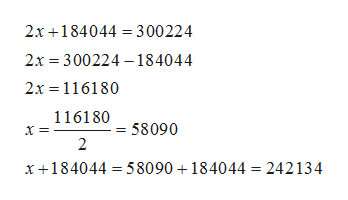The area of a state is 184,044 square miles greater than that of a second state. Their combined area is 300,224 square miles. What is the area of each state?

Question

The area of a state is 184,044 square miles greater than that of a second state. Their combined area is 300,224 square miles. What is the area of each state?

Step 1

Let the area of two states are x square miles and (x+184,044) square miles. Then according to given condition , their sum is 300,224 square miles.

Step 2

On solving the equation , we get th...help_outlineImage Transcriptionclose2x 184044 300224 2х %3D 300224 -184044 2x 116180 11618058090 х 2 x184044 58090+ 184044 = 242134 fullscreen

Want to see the full answer?

See Solution

Want to see this answer and more?

Our solutions are written by experts, many with advanced degrees, and available 24/7

See Solution
Tagged in

Equations and In-equations